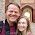## Monday, September 29, 2014

### Julia: Random Number Generator Functions

In this post I will explore the built in Random Number functions in Julia. These can be found in the documentation at: #random-numbers

As with most random number generators it is useful to manually set the 'seed'. This allows for replication of results across multiple runs.

Set seed is accomplished with the simple 'srand' function:

# We can verify that setting the seed results in identical draws from
# the random distribution by using the uniform [0,1) random draw
# function 'rand':

srand(123)
rand()
# 0.7684476751965699

srand(123); rand()
# 0.7684476751965699

# Julia random drawn objects have the convience of shaping themselves
# into random arrays the size specified by their arguments.
# For example a 2 by 10 random array

a1 = rand(2,10)
# 2x10 Array{Float64,2}:
# 0.940782  0.790201  0.900925  0.031831  …  0.759755  0.234544   0.627093
# 0.48      0.356221  0.529253  0.900681     0.19178   0.0976698  0.946697

# You can also use Julia to modify an existing array by filling it with
# random elements.
# First create a 3 x 3 of zeros

z1 = zeros(3,3)
# 3x3 Array{Float64,2}:
# 0.0  0.0  0.0
# 0.0  0.0  0.0
# 0.0  0.0  0.0

# Now populate it with random uniforms

rand!(z1)
# Notice the ! notation for functions that modify their arguments

z1
# 3x3 Array{Float64,2}:
# 0.615666  0.76537    0.256698
# 0.984495  0.322722   0.0808458
# 0.775836  0.0696626  0.275759

# rand can also be used to draw elements from the range of r in rand(r)
# For example, to draw a 2 x 2 array with elements drawn from 1 to 10.

rand(1:10,2,2)
# 2x2 Array{Int64,2}:
# 10  3
#  9  9

# We might also want to generate random boolean values which could be
# accomplished with either

rand(0:1,2,2).==1
#  true  false
# false   true
# Or the specific function

randbool(2,2)
#  false  false
#   true  false

# The final type of random variable that comes with the base
# install of Julia is the random normal generator:

randn(3,2)
# 3x2 Array{Float64,2}:
# -1.51181    0.139519
# -0.21379   -0.30305
# -0.711524  -0.048655

# This generates based on standard normal but of course we can easily
# any standard normal to have standard deviation x and mean y

x = 90
y = -34
randn(3,2)*x+y
# 3x2 Array{Float64,2}:
# -4.43119   -101.457
# -137.832    38.9093
# -9.66817   -20.2061

# In the original version of the post I mentioned that the base package did not contain much options regarding distributions to draw from. However, there is a package called distributions which I will explore more in depth in a future post which I have been promised can satisfy all of my distributional desires (see comments below).

1.using Distributions

z1 = zeros(3,3)

rand!(Gamma(1),z1)

1.I get the error: Gamma not defined

2.You must have missed the "using Distributions" this time. Gamma has always been exported:
julia> z1 = zeros(3,3)
3x3 Array{Float64,2}:
0.0 0.0 0.0
0.0 0.0 0.0
0.0 0.0 0.0

julia> rand!(Gamma(1),z1)
3x3 Array{Float64,2}:
0.139214 1.07667 0.00656263
2.52101 1.41252 0.152202
1.16747 0.20847 3.87497

3.Did you install Distributions with Pkg.add("Distributions") and load it with using Distributions?

4.Thanks still getting the basics of Julia :)

2.As Anonymous indicates, there is a very mature Distributions.jl package with every kind of distribution RNG you could possibly want.

1.3.julia> using Distributions

julia>

julia> z1 = zeros(3,3)
3x3 Array{Float64,2}:
0.0 0.0 0.0
0.0 0.0 0.0
0.0 0.0 0.0

julia>

julia> rand!(Gamma(1),z1)
3x3 Array{Float64,2}:
0.137952 4.78197 0.266333
1.64758 0.132392 0.909628
0.318518 0.488298 0.903232

1.